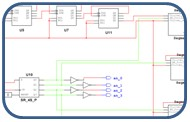# Teaching Digital Logic Fundamentals - Main Page

## Overview

Taking a hands on approach to learning digital logic can be difficult without the need to teach complex hardware descriptive languages (eg. VHDL). Multisim Programmable Logic Diagram (PLD) along with support for leading Digilent Teaching hardware allow educators to teach students the theory whilst students put it into practise. The PLD schematic allows educators and students to create graphical logic diagrams and deploy these to Digilent educational boards. In this tutorial series we demonstrate how digtal logic theory can be taught using educational hardware to provide a hands on approach to learning.

## Getting Started with Digilent Boards in Multisim

Getting Started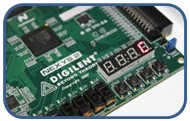Digilent provides a range of teaching boards which are supported in Multisim. This tutorial will explain how the simple process of creating logic diagrams with hardware inputs and outputs.

## Digital Logic Fundamentals

Logic Fundamentals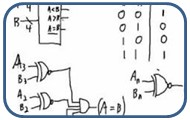Logic gates are the fundamentals of all digital designs. This tutorial will describe how students can learn this core knowledge through practical exploration.

## Logic Simplification

Logic Simplification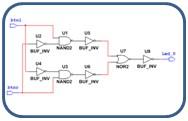This tutorial illustrates how we can take a digital schematic and simplify it using different logic gates achieving the same result when applied to hardware.

## Binary Comparison

Binary Comparator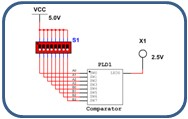This tutorial demonstrates how students can learn the process of comparing two binary strings through simulation and then through a hands-on approach using Digilent hardware.